Acoustics and Vibration Animations Daniel A. Russell, Ph.D. Graduate Program in Acoustics The Pennsylvania State University All text and images on this page are ©2004-2011 by Daniel A. Russell and may not used in other web pages or reports without permission.# The Vibration of a Fixed-Fixed String

### The natural modes of a fixed-fixed string

When the end of a string is fixed, the displacement of the string at that end must be zero. A transverse wave travelling along the string towards a fixed end will be reflected in the opposite direction. When a string is fixed at both ends, two waves travelling in opposite directions simply bounce back and forth between the ends.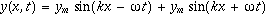The vibrational behavior of the string depends on the frequency (and wavelenth) of the waves reflecting back and forth from the ends.

A string which is fixed at both ends will exhibit strong vibrational response only at the resonance frequncies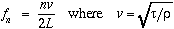is the speed of transverse mechanical waves on the string, L is the string length, and n is an integer. At any other frequencies, the string will not vibrate with any significant amplitude. The resonance frequencies of the fixed-fixed string are harmonics (integer multiples) of the fundamental frequency (n=1).

The vibrational pattern (mode shape) of the string at resonance will have the form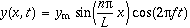.
This equation represents a standing wave. There will be locations on the string which undergo maximum displacement (antinodes) and locations which to not move at all (nodes). In fact, the string may be touched at a node without altering the string vibration. The animation below shows the vibration of a fixed-fixed string in its first four resonant modes.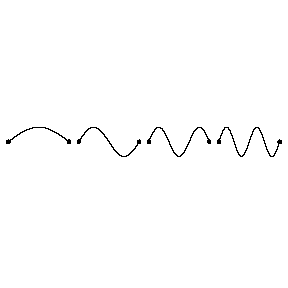### The vibration of fixed-fixed string plucked at a distance 1/3 of the length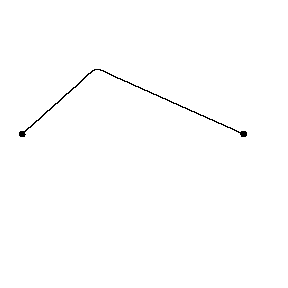When a fixed-fixed string is set into vibration by plucking it, the string will vibrate at many of its natural resonance frequencies at the same time. Exacly which resonance frequencies (and thus which mode shapes) make up the final string vibration depends on the shape of the initial string displacement. The movie at left (0.58MB mpeg) shows the vibration of a fixed-fixed string which has been plucked at a point 1/3 of its length from the left end (x = 1/3L). As you observe the vibration you should be able to visualize two wave pulses, one travelling clockwise, the other travelling counterclockwise. The time for one complete trip equals one period - if the string wave vibrating with a fundamental frequency of 440 Hz, then this vibration cycle would repeat itself 440 times per second.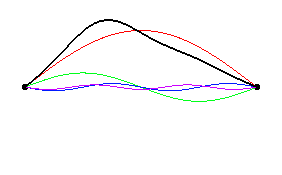Fourier's theorem says that any periodic function f(t) may be constructed from a combination of sin(w t) and cos(w t) functions with appropriate amplitudes and frequencies. The picture at left shows the amplitude and phase of the first 6 string modes which add up to produce the initial string position (plucked at x = 1/3L). [color code: sum, n=1, n=2, n=3, n=4, n=5, n=6 ] You should notice that modes corresponding to n=3 and n=6 are missing. This is because standing wave patterns which have a node at the pluck point will not be excited. Since any mode which is an integer multiple of n=3 will have a node at position x=L/3 these standing wave patterns will not be excited.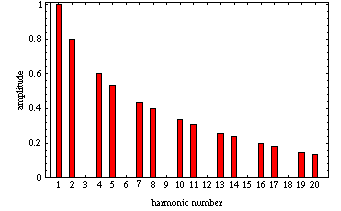A "frequency spectrum" may be constructed for this plucked string vibration by determining the standing wave mode number (resonance frequency) and amplitude for all mode shapes which are present in a given string vibraiton. The figure at left shows the mode number spectrum for the string struck at (plucked at x = 1/3L). Notice that all multiples of 3 are missing.

```
```

Back to the Vibrations and Waves: Demos and Animations Page